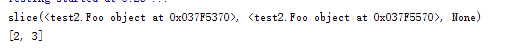﻿ python魔法方法-属性转换和类的表示详解_python_脚本之家
pythonpython魔法方法-属性转换和类的表示详解

•__int__(self)

•转换成整型，对应int函数。

•__long__(self)

•转换成长整型，对应long函数。

•__float__(self)

•转换成浮点型，对应float函数。

•__complex__(self)

•转换成 复数型，对应complex函数。

•__oct__(self)

•转换成八进制，对应oct函数。

•__hex__(self)

•转换成十六进制，对应hex函数。

•__index__(self)

•首先，这个方法应该返回一个整数，可以是int或者long。这个方法在两个地方有效，首先是 operator 模块中的index函数得到的值就是这个方法的返回值，其次是用于切片操作，下面会专门进行代码演示。

•__trunc__(self)

•当 math.trunc(self) 使用时被调用.__trunc__返回自身类型的整型截取 (通常是一个长整型).

•__coerce__(self, other)

•实现了类型的强制转换，这个方法对应于 coerce 内建函数的结果（python3.0开始去掉了此函数，也就是该魔法方法也没意义了，至于后续的版本是否重新加入支持，要视官方而定。）

•这个函数的作用是强制性地将两个不同的数字类型转换成为同一个类型，例如：class Foo(object):
def __init__(self, x):
self.x = x

def __int__(self):
return int(self.x) + 1

def __long__(self):
return long(self.x) + 1

a = Foo(123)
print int(a)
print long(a)
print type(int(a))
print type(long(a))def __int__(self):
return str(self.x)def __int__(self):
return list(self.x)class Foo(object):
def __init__(self, x):
self.x = x

def __int__(self):
return long(self.x) + 1

def __long__(self):
return int(self.x) + 1

a = Foo(123)
print int(a)
print long(a)
print type(int(a))
print type(long(a))__index__(self)：

import operator

class Foo(object):
def __init__(self, x):
self.x = x

def __index__(self):
return self.x + 1

a = Foo(10)
print operator.index(a)class Foo(object):
def __init__(self, x):
self.x = x

def __index__(self):
return 3

a = Foo('scolia')
b = [1, 2, 3, 4, 5]
print b[a]
print bclass Foo(object):
def __init__(self, x):
self.x = x

def __index__(self):
return int(self.x)

a = Foo('1')
b = Foo('3')
c = [1, 2, 3, 4, 5]
print c[a:b]a = Foo('1')
b = Foo('3')
c = slice(a, b)
print c
d = [1, 2, 3, 4, 5]
print d[c]

__coerce__(self, other)：

class Foo(object):
def __init__(self, x):
self.x = x

def __coerce__(self, other):
return self.x, str(other.x)

class Boo(object):
def __init__(self, x):
self.x = x

def __coerce__(self, other):
return self.x, int(other.x)

a = Foo('123')
b = Boo(123)
print coerce(a, b)
print coerce(b, a)总结：是调用了第一个参数的魔法方法。

•__str__(self)

•定义当 str() 被你的一个类的实例调用时所要产生的行为。因为print默认调用的就是str()函数。

•__repr__(self)

•定义当 repr()  被你的一个类的实例调用时所要产生的行为。 str() 和 repr() 的主要区别是其目标群体。 repr() 返回的是机器可读的输出，而 str() 返回的是人类可读的。  repr() 函数是交换模式默认调用的

•函数。

•__unicode__(self)

•定义当 unicode() 被你的一个类的实例调用时所要产生的行为。 unicode() 和 str() 很相似，但是返回的是unicode字符串。注意，如果对你的类调用 str() 然而你只定义了 __unicode__() ，那么其将不会

•工作。你应该定义 __str__() 来确保调用时能返回正确的值，并不是每个人都有心情去使用unicode()。

•__format__(self, formatstr)

•定义当你的一个类的实例被用来用新式的格式化字符串方法进行格式化时所要产生的行为。例如， "Hello, {0:abc}!".format(a) 将会导致调用 a.__format__("abc") 。这对定义你自己的数值或字符串类型

•是十分有意义的，你可能会给出一些特殊的格式化选项。

•__hash__(self)

•定义当 hash()被你的一个类的实例调用时所要产生的行为。它必须返回一个整数，用来在字典中进行快速比较。

•请注意，实现__hash__时通常也要实现__eq__。有下面这样的规则：a == b 暗示着 hash(a) == hash(b) 。也就是说两个魔法方法的返回值最好一致。

•这里引入一个‘可哈希对象'的概念，首先一个可哈希对象的哈希值在其生命周期内应该是不变的，而要得到哈希值就意味要实现__hash__方法。而哈希对象之间是可以比较的，这意味着要实现__eq__或

•者__cmp__方法，而哈希对象相等必须其哈希值相等，要实现这个特性就意味着__eq__的返回值必须和__hash__一样。

•可哈希对象可以作为字典的键和集合的成员，因为这些数据结构内部使用的就是哈希值。python中所有内置的不变的对象都是可哈希的，例如元组、字符串、数字等；而可变对象则不能哈希，例如列表、

•字典等。

•用户定义的类的实例默认是可哈希的，且除了它们本身以外谁也不相等，因为其哈希值来自于 id 函数。但这并不代表 hash(a) == id(a)，要注意这个特性。

•__nonzero__(self)

•定义当 bool() 被你的一个类的实例调用时所要产生的行为。本方法应该返回True或者False，取决于你想让它返回的值。(python3.x中改为__bool__)

•__dir__(self)

•定义当 dir() 被你的一个类的实例调用时所要产生的行为。该方法应该返回一个属性的列表给用户。

•__sizeof__(self)

•定义当 sys.getsizeof() 被你的一个类的实例调用时所要产生的行为。该方法应该以字节为单位，返回你的对象的大小。这通常对于以C扩展的形式实现的Python类更加有意义，其有助于理解这些扩展。